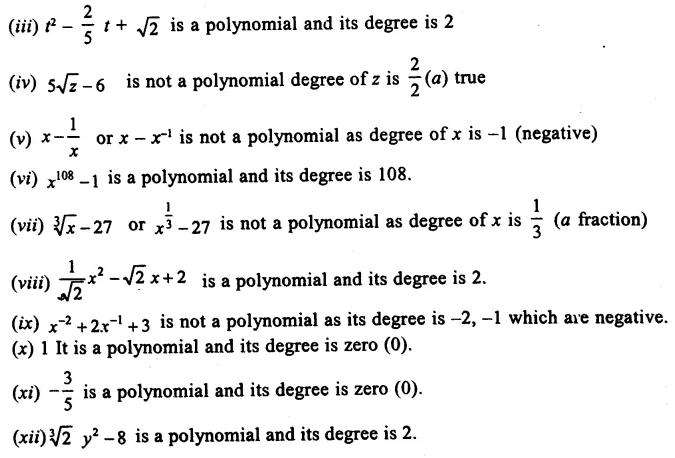## RS Aggarwal Class 9 Solutions Chapter 2 Polynomials Ex 2A

These Solutions are part of RS Aggarwal Solutions Class 9. Here we have given RS Aggarwal Solutions Class 9 Chapter 2 Polynomials Ex 2A.

Other Exercises

Question 1.
Solution:
(i) x5-2x3+x+7 is a polynomial and its degree is 5
(ii) y3-√3y is a polynomial and its degree is 3Question 2.
Solution:
(i) Degree is 1
(ii) degree is 3
(iii) degree is zero
(iv) degree is 7
(v) degree is 10
(vi) degree is 2

Question 3.
Solution:
(i) Coefficient of x3 is -5.
(ii) Coefficient of x is -2√2
(iii) Coefficient of x2 is $$\frac { \pi }{ 3 }$$
(iv) Coefficient of x2 is 0

Question 4.
Solution:
(i) Example of a binomial of degree 27 is 4x27 – 5
(ii) Example of a monomial of degree 16 is x16
(iii) Example of trinomial of degree 3 is 2x3 + 7x + 4

Question 5.
Solution:
(i) 2x2 + 4x is a quadratic polynomial. (Degree 2)
(ii) x – x3 is a cubic polynomial (Degree 3)
(iii) 2 – y – y2 is a quadratic polynomial (Degree 2)
(iv) – 7 + z is a linear polynomial (Degree 1)
(v) 5t is a linear polynomial (Degree 1)
(vi) p3 is a cubic polynomial (Degree 3)

Hope given RS Aggarwal Solutions Class 9 Chapter 2 Polynomials Ex 2A are helpful to complete your math homework.

If you have any doubts, please comment below. Learn Insta try to provide online math tutoring for you.